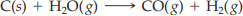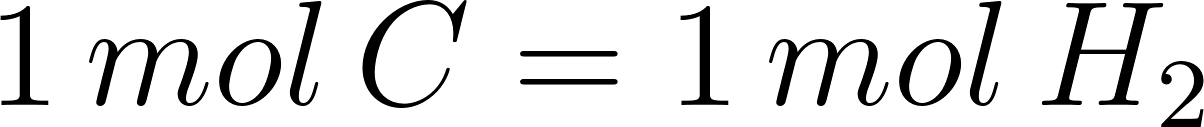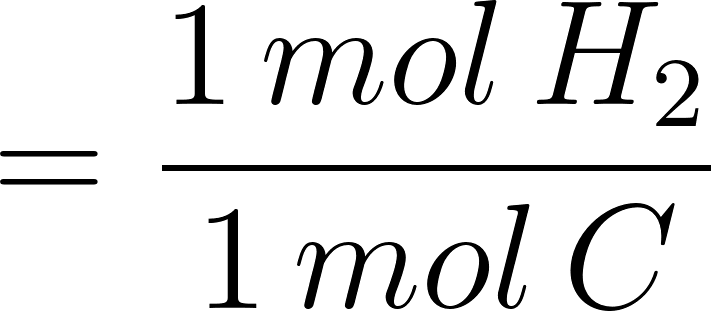×
Get Full Access to Introductory Chemistry - 5 Edition - Chapter 11 - Problem 89p
Get Full Access to Introductory Chemistry - 5 Edition - Chapter 11 - Problem 89p

×

# Consider the chemical reaction:C(s) + H2O(g) ? CO(g) + H2OHow many liters of hydrogenISBN: 9780321910295 34

## Solution for problem 89P Chapter 11

Introductory Chemistry | 5th Edition

• Textbook Solutions
• 2901 Step-by-step solutions solved by professors and subject experts
• Get 24/7 help from StudySoup virtual teaching assistantsIntroductory Chemistry | 5th Edition

4 5 1 342 Reviews
17
2
Problem 89P

PROBLEM 89P

Consider the chemical reaction:

C(s) + H2O(g) → CO(g) + H2O

How many liters of hydrogen gas are formed from the complete reaction of 1.07 mol of C? Assume that the hydrogen gas is collected at 1.0 atm and 315 K.

Step-by-Step Solution:

Problem 89P

Consider the chemical reaction:How many liters of hydrogen gas are formed from the complete reaction of 1.07 mol of C? Assume that the hydrogen gas is collected at 1.0 atm and 315 K.

Step by Step Solution

Step 1 of 3

The given chemical reaction is as follows.From the above reaction, one mole of carbon reacts with one mole of water.

Hence, the conversion factor is;Step 2 of 3

Step 3 of 3

##### ISBN: 9780321910295

Unlock Textbook Solution